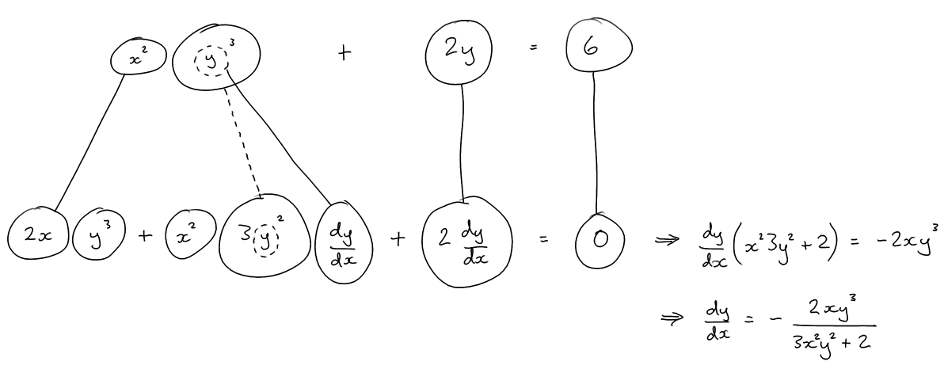# Thread: Implicit differentiation

1. ## Implicit differentiation

Hello,

i have a problem

x^2y^3+2y=6 solve using implicit differentiation.

so am i suppose to take the derivative first?

which would be 3x^2y^2+2xy^3-2=0?

2. ## Re: Implicit differentiation

Hey arcticreaver.

What exactly are you trying to solve?

3. ## Re: Implicit differentiation

oh sorry, find dy/dx using implicit differentiation

4. ## Re: Implicit differentiation

Ok that makes more sense.

Remember two rules: d/dx f(x) = f'(x) and d/dx f(u(x)) = u'(x)*f'(u(x)) (let u(x) = y). The rest includes the product, quotient and power rules.

5. ## Re: Implicit differentiation

can anyone work out the problem?

6. ## Re: Implicit differentiationOriginally Posted by arcticreavercan anyone work out the problem?
Yes we can. The question is, can you? That's the whole point of the site, to give you guidance so that YOU can solve your problems.

If the answer to that question is no, then what have you tried and where are you stuck?

7. ## Re: Implicit differentiation

We are given the implicit relationship:

$\displaystyle x^2y^3+2y=6$

Now, using the product and chain rules, we find:

$\displaystyle x^2\left(3y^2\frac{dy}{dx} \right)+2x(y^3)+2\frac{dy}{dx}=0$

Now, solve for $\displaystyle \frac{dy}{dx}$

8. ## Re: Implicit differentiation

thanks for writing that out MarkFL2.

dy/dx = -2/ x^2(6y^2)+2xy^3?

9. ## Re: Implicit differentiation

That's incorrect...in general you want to arrange with the terms having dy/dx as a factor on one side and the other terms on the other. Then factor out dy/dx, and divide through by the other factor to isolate dy/dx. Give this another try...

10. ## Re: Implicit differentiation

okay so if i am understanding this correctly, it would be - x^2(6y^2)+2 / 2x(y^3)?

11. ## Re: Implicit differentiation

Let's go back to:

$\displaystyle x^2\left(3y^2\frac{dy}{dx} \right)+2x(y^3)+2\frac{dy}{dx}=0$

Clean this up some:

$\displaystyle 3x^2y^2\frac{dy}{dx}+2xy^3+2\frac{dy}{dx}=0$

Arrange with dy/dx terms on the left, all else on the right:

$\displaystyle 3x^2y^2\frac{dy}{dx}+2\frac{dy}{dx}=-2xy^3$

Factor out dy/dx on the left side:

$\displaystyle \frac{dy}{dx}(3x^2y^2+2)=-2xy^3$

Divide through by $\displaystyle 3x^2y^2+2$

$\displaystyle \frac{dy}{dx}=-\frac{2xy^3}{3x^2y^2+2}$

12. ## Re: Implicit differentiation

Just in case a picture helps (and otherwise to correct a small slip/typo, in Mark's differentiation of y^3)...... where (key in spoiler) ...

Spoiler:... is the chain rule. Straight continuous lines differentiate downwards (integrate up) with respect to the main variable (in this case x), and the straight dashed line similarly but with respect to the dashed balloon expression (the inner function of the composite which is subject to the chain rule).

But this is wrapped inside the legs-uncrossed version of...... the product rule, where, again, straight continuous lines are differentiating downwards with respect to x.

__________________________________________________ __________

Don't integrate - balloontegrate!

Balloon Calculus; standard integrals, derivatives and methods

Balloon Calculus Drawing with LaTeX and Asymptote!

#### Search Tags

differentiation, implicit